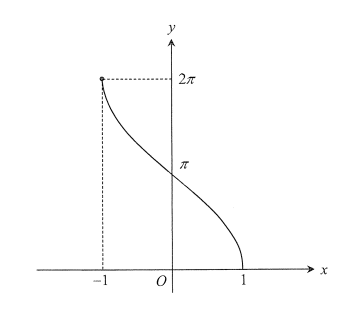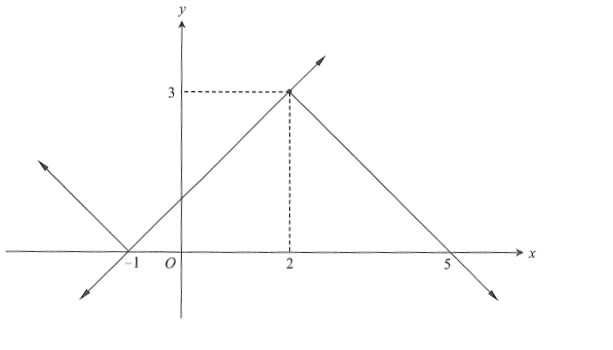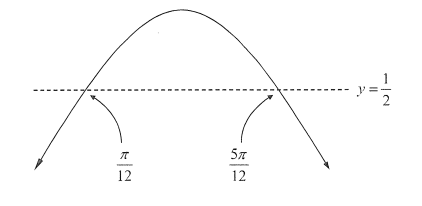# 2017 HSC Maths Ext 1 Exam Paper Solutions

In this post, we give you the solutions to the 2017 Maths Extension 1 paper.Have you seen the 2017 HSC Mathematics Extension 1 Paper, yet? In this post, we will work our way through the 2017 HSC Maths Extension 1 Exam Paper and give you the solutions, written by our leading teacher Jonathan Le, to all of the questions.

Read on, to see how to answer all of the 2017 questions.

## 2017 HSC Mathematics Extension 1 Exam Paper Solutions

This paper has two sections

• Section I consists of 10 Multiple Choice Questions
• Section II consists of 4 Extended Response Questions

## Section 1: 10 Multiple Choice Questions (10 Marks)

##### 1
A Use factor theorem, it is clear that, $$P(2)=0$$

so $$(x-2)$$ is a factor.

##### 2
B We have $$3\log_a(2)=1.893$$, so calculate $$\log_a(4)=2\log_a(2)$$.
##### 3
B $$\angle BAD = 180^\circ – 110^\circ = 70^\circ$$ (Opposite angles in a cyclic quadrilateral) and $$\angle TAB=65^\circ$$ (alternate segment theorem).
##### 4
C Standard Auxiliary angle formula problem.
##### 5
D As $$x\rightarrow \infty$$, $$y\rightarrow -2$$ The only graph with horizontal asymptote $$y=-2$$ is (D)
##### 6
D The normal is usually $$x+py=p^3+2p$$ for a point with co-ordinate $$(2p,p^2)$$ (Set $$a=1$$ in the formula in the reference sheet). Now replace $$p$$ with $$\frac{1}{p}$$ and re-arrange.
##### 7
AThe domain is found by solving $$-1\leq \frac{3}{x} \leq 1$$. The direction of the arrows can be found quickly by drawing $$y=\frac{3}{x}$$ and the lines $$y=\pm 1$$
##### 8
C Use the chain rule $$\frac{dA}{dt}=\frac{dA}{dr}\times\frac{dr}{dt}=2\pi r \times 5$$, then substitute in $$r=15$$
##### 9
C Find the power of $$x$$ of each general term. Option (C) yields $$7r-28$$, and is actually the only one that yields an integer value of $$r$$.
##### 10
BThe total number of ways to cross 2 squares out of 9 is $$^9C_3$$. Since the grid is small, we can manually count the number of possible ways to get three in a row, which will be 8. Hence, the probability is $$\frac{8}{^9C_3}$$.

## Section 2:  Extended Response Questions (60 Marks)

### Question 11 (15 marks)

(a)

\begin{equation*}
x_p=\frac{3(-4)+2(1)}{2+3}=-2
\end{equation*}

(b)

\begin{align*}
\frac{d}{dx}\big(\tan^{-1}(x^3)\big)&=\frac{3x^2}{1+(x^3)^2}\\
&=\frac{3x^2}{1+x^6}
\end{align*}

(c)

Multiply both sides by $$(x+1)^2$$.
\begin{align*}
\frac{2x}{x+1} &> 1\\
2x(x+1) &> (x+1)^2\\
(x+1)^2-2x(x+1)&<0\\
(x+1)[x+1-2x]&<0\\
(x+1)(1-x)&<0\\
(x+1)(x-1)&>0
\end{align*}

Hence, $$x>1$$ or $$x<-1$$

(d)

Domain:  $$-1\leq x \leq 1$$

Range: $$0 \leq y \leq 2\pi$$(e)

Compute the differential term first.

\begin{align*}
x&=u^2-1\\
dx&=2u~du
\end{align*}

Compute the new limits, choosing u to be positive.

\begin{align*}
x=3 ~~~\rightarrow ~~~& u=2\\
x=0 ~~~\rightarrow ~~~& u=1\\
\end{align*}

Substitute.

\begin{align*}
\int_0^3 \frac{x}{\sqrt{x+1}}dx&=\int_1^2\frac{u^2-1}{u}\times 2u~du\\
&= 2\int_1^2 u^2-1 du\\
& = 2\Big[\frac{u^3}{3}-u\Big]_1^2\\
&= \frac{8}{3}
\end{align*}

(f)

Use the fact that $$\int f^n(x)f'(x)dx=\frac{1}{n+1}f^{n+1}(x)+C$$

\begin{equation*} \int \sin^2x\cos x dx=\frac{1}{3} \sin^3 x + C\end{equation*}

(g) (i)

\begin{equation*}P(3 \text{ red})=\binom{8}{3}\Big(\frac{1}{5}\Big)^3\Big(\frac{4}{5}\Big)^5\end{equation*}

(g) (ii)

\begin{equation*}P(\text{no flowers})=\Big(\frac{4}{5}\Big)^8\end{equation*}

(g) (iii)

\begin{align*}
P(\text{at least one red}) &= 1-P(\text{no red})\\
& = 1-\Big(\frac{4}{5}\Big)^8
\end{align*}

### Question 12 (15 marks)

(a)

Let $$D$$ be any point on the major arc $$AC$$.

\begin{align*}
\angle ADC &= 50^\circ~~~(\angle \text{ at centre } =2\times \angle \text{ at circumference})\\
\\
\angle ABC&= 180^\circ – 50^\circ~~~(\text{opposite angles of a cyclic quadrilateral are supplementary})\\
& = 30^\circ
\end{align*}

(b) (i)

The graph of $$y=|x+1|$$ is the shape of $$y=|x|$$ but shifted to the left by 1 unit.

The graph of $$y=3-|x-2|$$ is the shape of $$y=|x|$$ but upside-down, shifted to the right by 1 unit and shifted up by 3 units, so the vertex is at $$(2,3)$$. But observe that $$(2,3)$$ lies on the right branch of $$y=|x+1|$$.(b) (ii)

We are asked to solve $$|x+1| + |x-2| = 3$$, but this is equivalent to $$|x+1|=3-|x-2|$$, which in turn is equivalent to solving $$y=|x+1|$$ and $$y=3-|x-2|$$ simultaneously. Since we have the graphs of the two equations above, we can find the solutions geometrically.

From the diagram above, the two equations are equal over the domain $$-1\leq x \leq 2$$, so therefore that must be the solution set to the original equation $$|x+1|+|x-2|=3$$.

(c) (i)

First, find the ratio of the left part to the right part.

\begin{align*}
\frac{V_{left}}{V_{right}}&=\frac{\pi\int_{-1}^h 1-x^2~dx}{\pi\int_h^1 1-x^2~dx}\\
&=\dfrac{\Big[x-\dfrac{x^3}{3}\Big]_{-1}^h}{\Big[x-\dfrac{x^3}{3}\Big]_h^1}\\
&= \frac{h-\frac{h^3}{3}+\frac{2}{3}}{\frac{2}{3}-h+\frac{h^3}{3}}\\
& = \frac{3h-h^3+2}{2-3h+h^3}
\end{align*}

But the ratio is given to be $$2:1$$, so this fraction must be equal to 2.

\begin{align*}
\frac{3h-h^3+2}{2-3h+h^3}&=2\\
3h-h^3+2&=2(2-3h+h^3)\\
3h-h^3+2&=4-6h+2h^3
\end{align*}

And hence the required result $$3h^3-9h+2=0$$.

(c) (ii)

Define $$f(h)=3h^3-9h+2$$, so $$f'(h)=9h^2-9$$.

\begin{align*}
h_2&=h_1-\frac{f(h_1)}{f'(h_1)}\\
&=h_1 – \frac{3h_1^3-9h_1+2}{9h_1^2-9}\\
&=\frac{2}{9}~~~(\text{since } h_0=1)
\end{align*}

(d)

Differentiate $$t$$ with respect to $$x$$ and notice that $$\frac{dt}{dx}=\frac{1}{dx/dt}=\frac{1}{v}$$.

\begin{align*}
\frac{dt}{dx}&=2e^{-2x}\\
\frac{1}{v}&= 2e^{-2x}\\
v&=\frac{1}{2}e^{2x}\\
v^2&=\frac{1}{4}e^{4x}
\end{align*}

But $$a=\frac{d}{dx}\Big(\frac{1}{2}v^2\Big)$$.

\begin{align*}
a&=\frac{d}{dx}\big(\frac{1}{8}e^{4x}\big)\\
&=\frac{1}{2}e^{4x}
\end{align*}

(e)

\begin{align*}
\lim_{x \rightarrow 0} \Big(\frac{1-\cos2x}{x^2}\Big)&=\lim_{x \rightarrow 0} \Big(\frac{2\sin^2x}{x^2}\Big)\\
&=2\times \lim_{x \rightarrow 0} \Big(\frac{\sin x}{x} \times \frac{\sin x}{x}\Big)\\
&=2\times \lim_{x \rightarrow 0} \Big(\frac{\sin x}{x} \Big)\times \lim_{x \rightarrow 0} \Big(\frac{\sin x}{x} \Big)\\
&=2\times 1 \times 1\\
&=2
\end{align*}

### Question 13 (15 marks)

(a)

The particle satisfies the equation for simple harmonic motion $$v^2=n^2(a^2-x^2)$$.

Substitute in the given data and solve simultaneously.

\begin{align*}
x=2,v=4 ~~&\rightarrow~~ 16=n^2(a^2-4)\\
x=5,v=3 ~~&\rightarrow~~ 9=n^2(a^2-25)
\end{align*}

Divide these equations.

\begin{align*}
\frac{16}{9} &= \frac{a^2-4}{a^2-25}\\
16a^2-400&=9a^2-36\\
7a^2&=364\\
a^2&=52
\end{align*}

Substitute back in to find $$n$$.

\begin{align*}
9&=n^2(52-25)\\
n^2&=\frac{9}{27}\\
n^2&=\frac{1}{3}\\
n&=\frac{1}{\sqrt{3}}~~~(\text{taking } n>0)
\end{align*}

Hence, the period is $$T=\frac{2\pi}{n}=2\pi\sqrt{3}$$.

(b) (i)

$$(1+x)^n+(1-x)^n=\Big[\binom{n}{0}+\binom{n}{1}x+\binom{n}{2}x^2 + \cdots + \binom{n}{n}x^n\Big] +\Big[\binom{n}{0}-\binom{n}{1}x+\binom{n}{2}x^2 – \cdots + (-1)^n\binom{n}{n}x^n\Big]$$

Notice that all the odd powered terms cancel and that we have two of each even powered term. Furthermore, the last term does not cancel because $$n$$ is even.

Hence, we have the expansion

\begin{align*}
(1+x)^n+(1-x)^n &= 2\binom{n}{0}+2\binom{n}{2}x^2+2\binom{n}{4}x^4+\cdots + 2\binom{n}{n}x^n\\
&= 2\Big[\binom{n}{0}+\binom{n}{2}x^2+\binom{n}{4}x^4+\cdots + \binom{n}{n}x^n\Big]
\end{align*}

(b) (ii)

Differentiate both sides of (i) with respect to $$x$$.

\begin{equation*}n(1+x)^{n-1}-n(1-x)^{n-1}=2\Big[2\binom{n}{2}x+4\binom{n}{4}x^3+\cdots + n\binom{n}{n}x^{n-1}\Big]\end{equation*}

(b) (iii)

Let $$x=1$$ and then re-arrange.

\begin{align*}
2\Big[2\binom{n}{2}+4\binom{n}{4}+ \cdots + n\binom{n}{n}\Big]&=n2^{n-1}\\
2\binom{n}{2}+4\binom{n}{4}+ \cdots + n\binom{n}{n}=n2^{n-2}\\
\binom{n}{2}+2\binom{n}{4}+ \cdots + \frac{n}{2}\binom{n}{n}=n2^{n-3}
\end{align*}

(c) (i)

Let $$y=0$$ to find the time of flight.

\begin{align*}
Vt\sin\theta-\frac{1}{2}gt^2&=0\\
V\sin\theta-\frac{1}{2}gt&=0\\
t&=\frac{2V\sin\theta}{g}
\end{align*}
Substitute back into $$x$$ to find horizontal range.
\begin{align*}
x&= V\Big(\frac{2V\sin\theta}{g}\Big)\cos\theta\\
&= \frac{2V^2\sin\theta\cos\theta}{g}\\
&= \frac{V^2\sin2\theta}{g}\\
\end{align*}

(c) (ii)

Substitute the given inequality for $$V^2$$ into (i).
\begin{align*}
x & = \frac{V^2\sin2\theta}{g}\\
&< \frac{100g\sin2\theta}{g}\\
&=100\sin2\theta\\
&\leq100 ~~~ (\text{ since } \sin2\theta\leq1)
\end{align*}
Hence we have the bound $$x<100.$$

(c) (iii)

We are given that $$\frac{V^2\sin2\theta}{g}\geq 100$$

Let $$V^2=200g$$.

\begin{align*}
\frac{200g\sin2\theta}{g}&\geq 100\\
200\sin2\theta &\geq 100\\
\sin2\theta \geq \frac{1}{2}
\end{align*}
The boundary of the inequality is obtained by solving $$\sin2\theta = \frac{1}{2}$$
\begin{align*}
\sin2\theta&=\frac{1}{2}\\
2\theta &= \frac{\pi}{6},\frac{5\pi}{6}\\
\theta &= \frac{\pi}{12},\frac{5\pi}{12}
\end{align*}

A rough sketch shows that the values of $$\theta$$ must lie between these two boundaries, since we want the sine curve to be above the line $$y=\frac{1}{2}$$. Hence, we have the result $$\frac{\pi}{12} \leq \theta \leq \frac{5\pi}{12}$$(c) (iv)

The greatest height is achieved when $$\dot{y}=0.$$

\begin{align*}
V\sin\theta-gt&=0\\
t &= \frac{V\sin\theta}{g}
\end{align*}
For the actual maximum height, substitute this into $$y$$.
\begin{align*}
y_{max}&=Vt\sin\theta-\frac{1}{2}gt^2\\
& = V\Big(\frac{V\sin\theta}{g}\Big)\sin\theta-\frac{1}{2}g\Big(\frac{V\sin\theta}{g}\Big)^2\\
& = \frac{V^2\sin^2\theta}{g}-\frac{V^2\sin^2\theta}{2g}\\
& = \frac{V^2\sin^2\theta}{2g}\\
& = \frac{200g\sin^2\theta}{2g} ~~~~ (\text{since } V^2=200g)\\
& = 100\sin^2\theta
\end{align*}

But note that $$\frac{\pi}{12} \leq \theta \leq \frac{5\pi}{12}$$, so the maximum height is attained when $$\theta=\frac{5\pi}{12}$$ (the curve is still increasing at this stage and has not yet dipped down).

Hence the maximum height is

\begin{align*}
y_{max} &=100\sin^2\big(\frac{5\pi}{12}\big)\\
& = 50 – 50\cos\Big(\frac{5\pi}{6}\Big)\\
& = 50 + 25 \sqrt{3}
\end{align*}

Note: Given that this part was only worth 2 marks, it most likely wasn’t necessary to go as far as finding a nice exact form for $$y_{max}$$. Full marks most likely awarded for finding that $$y_{max}=100\sin^2\Big(\frac{5\pi}{12}\Big)$$.

### Question 14 (15 Marks)

(a)

Base Case: test $$n=1$$

\begin{equation*}8^3+6^1=518=7\times 74\end{equation*}

Hence, the result is true for $$n=1$$

Inductive Hypothesis: assume true for $$n=k$$

$$8^{2k+1}+6^{2k-1}=7M~~~ (\text{where } M \text{ is an integer})$$

Inductive Step: prove true for $$n=k+1$$

\begin{align*}
8^{2(k+1)+1}+6^{2(k+1)-1}&=8^{2k+3}+6^{2k+1}\\
&=8^2\times 8^{2k+1}+6^2\times 6^{2k-1}\\
& = 8^2\times (7M-6^{2k-1})+6^2\times 6^{2k-1}\\
& = 64 \times 7M – 64(6^{2k-1})+36(6^{2k-1})\\
& = 64 \times 7M – 28(6^{2k-1})\\
& = 7\big[64M-4(6^{2k-1})\big]
\end{align*}

And hence, the result is true by the principle of mathematical induction.

(b) (i)

The equation of the tangent from $$P$$ is $$y=px-p^2$$ (reference sheet), noting that $$a=1$$. Solve simultaneously with the bottom parabola $$x^2=-4ay.$$

\begin{align*}
x^2&=-4a(px-p^2)\\
&=-4apx+4ap^2\\
x^2+4apx-4ap^2&=0
\end{align*}

(b) (ii)

Note that the roots of the equation in (i) are the $$x$$ coordinates of where the tangent intersects $$Q$$ and $$R$$.

\begin{align*}
x_M &= \frac{x_R+x_Q}{2}\\
&= \frac{\text{Sum of roots of the quadratic in (i)}}{2}\\
& = \frac{-4ap}{2}\\
& = -2ap
\end{align*}
Substitute back into the linear function to find the $$y$$ coordinate.
\begin{align*}
y_M&=p(-2ap)-p^2\\
&=-2ap^2-p^2\\
&=-p^2(2a+1)
\end{align*}

Hence, $$M$$ has coordinates $$M(-2ap,-p^2(2a+1))$$.

(b) (iii)

If $$M$$ lies on the parabola $$x^2=-4y$$, then it must satisfy that equation. So we substitute the coordinates of $$M$$ into the equation, then solve for $$a$$.

\begin{align*}
(-2ap)^2 &= -4(-p^2(2a+1))\\
4a^2p^2&=4p^2(2a+1)\\
a^2 &= 2a+1\\
a^2-2a-1&=0\\
a&=1\pm\sqrt{2}
\end{align*}

Since $$a>0$$, the value of $$a$$ is $$a=1+\sqrt{2}$$

(c) (i)

\begin{align*}
\frac{d}{dt}(F(t)e^{0.4t})&=F'(t)e^{0.4t}+0.4e^{0.4t}F(t)\\
&=(50e^{-0.5t}-0.4F(t))e^{0.4t}+0.4e^{0.4t}F(t)\\
&= 50e^{-0.1t}-0.4e^{0.4t}F(t)+0.4e^{0.4t}F(t)\\
&=50e^{-0.1t}
\end{align*}

(c) (ii)

Integrate the result from (i) with respect to $$t$$.

\begin{equation*}F(t)e^{0.4t}=-500e^{-0.1t}+C\end{equation*}

Substitute in the fact that $$F(0)=0$$ (given).

\begin{align*}
0\times e^0 &= -500e^0+c\\
C &= 500
\end{align*}

Hence

\begin{align*}
F(t)e^{0.4t}&=-500e^{-0.1t}+500\\
F(t)&=-500e^{-0.5t}+500e^{-0.4t}\\
&= 500(e^{-0.4t}-e^{-0.5t})
\end{align*}

(c) (iii)

\begin{align*}
F'(t)&=500(-0.4e^{-0.4t}+0.5e^{-0.5t})\\
&= -200e^{-0.4t}+250e^{-0.5t}\\
&= 0 ~~~~(\text{for max/min value of }F)
\end{align*}

Solve for $$t$$:

\begin{align*}
200e^{-0.4t} &=250e^{-0.5t}\\
200e^{0.1t}&= 250\\
e^{0.1t}&=\frac{5}{4}\\
t&=10\ln\Big(\frac{5}{4}\Big)
\end{align*}